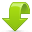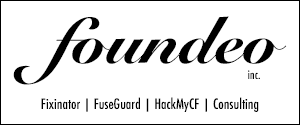# Implicit Structure Notation ColdFusion

Implicit Structure Notation was added to ColdFusion 8, this allows you to create a struct on the fly in one line.

Here's a simple example of how the new short hand notation works:

```<cfset person = { firstname="Pete", lastname="Freitag" }>
```

In Prior versions we might have done something like this, which is equivalent to the above code:

```<cfset person = StructNew()>
<cfset person.firstname = "Pete">
<cfset person.lastname = "Freitag">
```

As you can see the new shortcut notation is very handy!

### You might also like:Download FuseGuard WAF for ColdFusionPete,
I know this is an old thread, but let me know if you've ever run into this problem before and know WHY it's doing it.

Put this code in a CFM file and run it to see what it's doing.

<cfscript>
//testMethod(): testing the structure notation of CF
public any function testMethod(required any arg1, required struct arg2, string arg3){
return arguments;
}

goodStruct = StructNew();
goodStruct.var1 = DateFormat(Now(), 'mmm. d, yyyy');
goodStruct.var2 = testMethod(arg1=12, arg2={key1="value1",key2="value2"}, arg3="Good");
goodStruct.var3 = CreateODBCDateTime(Now());

if(1 == 1){
badStruct.var1 = DateFormat(Now(), 'mmm. d, yyyy');
}
</cfscript>

<cfdump var="#goodStruct#" label="Good Struct">
<p>The above struct is NOT created inside an if statement and thus works as expected.<br/></p>